Open in App
Not now

# Pygame – Drawing Objects and Shapes

• Difficulty Level : Expert
• Last Updated : 31 Oct, 2021

In this article, we are going to see how to draw an object using Pygame. There can be two versions for drawing any shape, it can be a solid one or just an outline of it.

## Drawing Objects and Shapes in PyGame

You can easily draw basic shapes in pygame using the draw method of pygame.

### Drawing Rectangle shape:

To draw a rectangle in your pygame project you can use draw.rect() function.

Syntax: pygame.draw.rect(surface, color, rect, width)

Parameters:

• surface :- Here we can pass the surface on which we want to draw our rectangle. In the above example, we created a surface object named ‘window’.
• color :- Here we can pass the color for our rectangle. We are using blue color in our example.
• rect :- Here we can pass the rectangle, position, and dimensions.
• width :- Here we can pass the line thickness. we can also create a solid rectangle by changing the value of this width parameter. So let’s look at that.

First, import the required module and initialize pygame. Now, Create the surface object of a specific dimension using the display.set_mode() method of pygame. Fill the background of the surface object with white color using the fill() function of pygame. Create a rectangle using the draw.rect() method of pygame. Update the Surface object.

Example 1: Drawing outlined rectangle using pygame.

## Python3

 `# Importing pygame module``import` `pygame``from` `pygame.``locals` `import` `*` `# initiate pygame and give permission``# to use pygame's functionality.``pygame.init()` `# create the display surface object``# of specific dimension.``window ``=` `pygame.display.set_mode((``600``, ``600``))` `# Fill the scree with white color``window.fill((``255``, ``255``, ``255``))` `# Using draw.rect module of``# pygame to draw the outlined rectangle``pygame.draw.rect(window, (``0``, ``0``, ``255``),``                 ``[``100``, ``100``, ``400``, ``100``], ``2``)` `# Draws the surface object to the screen.``pygame.display.update()`

Output :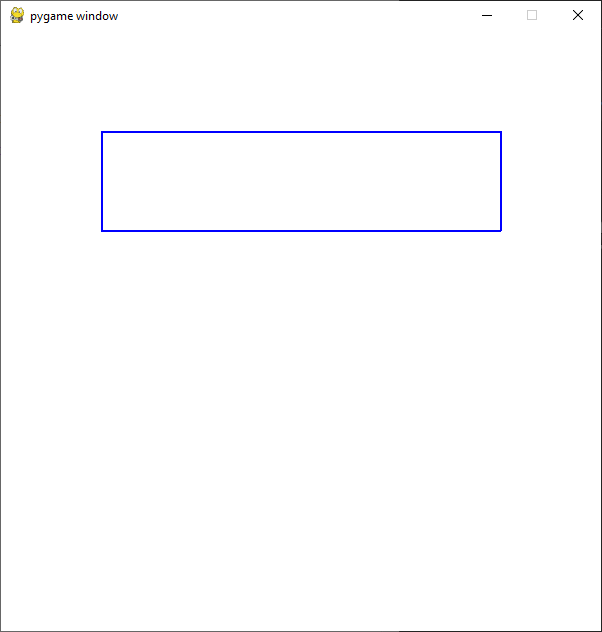We can create a solid rectangle by setting the width parameter equal to 0 and the rest of the approach remains the same.

Example 2: Drawing a solid rectangle.

## Python3

 `# Importing pygame module``import` `pygame``from` `pygame.``locals` `import` `*` `# initiate pygame and give permission``# to use pygame's functionality.``pygame.init()` `# create the display surface object``# of specific dimension.``window ``=` `pygame.display.set_mode((``600``, ``600``))` `# Fill the scree with white color``window.fill((``255``, ``255``, ``255``))` `# Using draw.rect module of``# pygame to draw the solid rectangle``pygame.draw.rect(window, (``0``,   ``0``, ``255``),``                 ``[``100``, ``100``, ``400``, ``100``], ``0``)` `# Draws the surface object to the screen.``pygame.display.update()`

Output :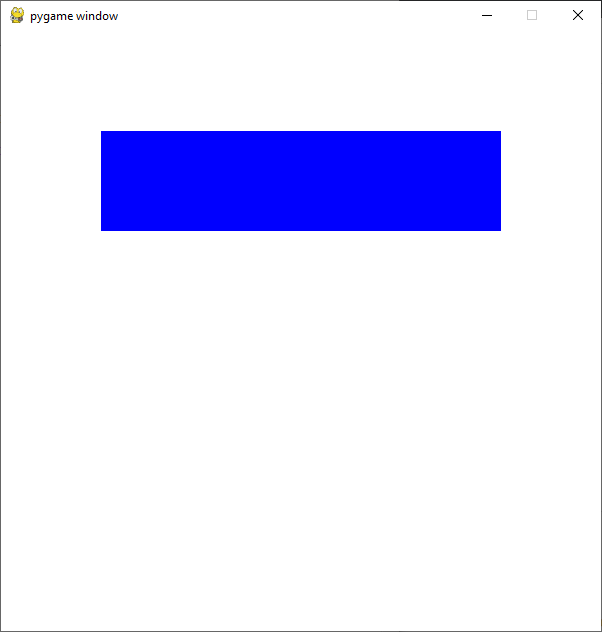### Drawing Circle Shape:

To draw a circle in your pygame project you can use draw.circle() function. The entire approach is the same as above only the function and parameters are changed accordingly.

Syntax : pygame.draw.circle(surface, color, center, radius, width)

Parameters :

• surface :- Here we can pass the surface on which we want to draw our circle. In the above example, we created a surface object named ‘window’.
• color :- Here we can pass the color for our circle. We are using green color in our example.
• center :- Here we can pass the ( x, y ) coordinates for the center of the circle.
• radius :- Here we can pass the radius of our circle.
• width :- Here we can pass the line thickness. we can also create a solid circle by changing the value of this width parameter. So let’s look at that.

Example 1: Drawing a hollow circle.

## Python3

 `# Importing pygame module``import` `pygame``from` `pygame.``locals` `import` `*` `# initiate pygame and give permission``# to use pygame's functionality.``pygame.init()` `# create the display surface object``# of specific dimension.``window ``=` `pygame.display.set_mode((``600``, ``600``))` `# Fill the scree with white color``window.fill((``255``, ``255``, ``255``))` `# Using draw.rect module of``# pygame to draw the solid circle``pygame.draw.circle(window, (``0``, ``255``, ``0``),``                   ``[``300``, ``300``], ``170``, ``3``)` `# Draws the surface object to the screen.``pygame.display.update()`

Output :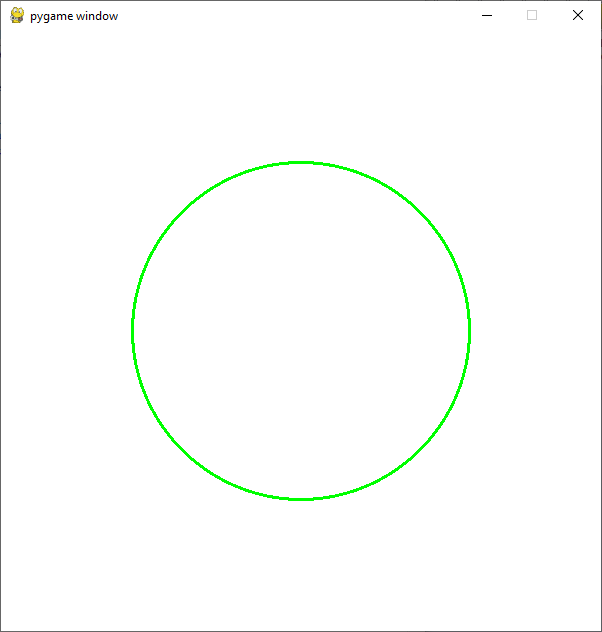We can create a solid circle by setting the width parameter equal to 0.

Example 2: drawing a solid circle

## Python3

 `# Importing pygame module``import` `pygame``from` `pygame.``locals` `import` `*` `# initiate pygame and give permission``# to use pygame's functionality.``pygame.init()` `# create the display surface object``# of specific dimension.``window ``=` `pygame.display.set_mode((``600``, ``600``))` `# Fill the scree with white color``window.fill((``255``, ``255``, ``255``))` `# Using draw.rect module of``# pygame to draw the solid circle``pygame.draw.circle(window, (``0``, ``255``, ``0``),``                   ``[``300``, ``300``], ``170``, ``0``)` `# Draws the surface object to the screen.``pygame.display.update()`

Output :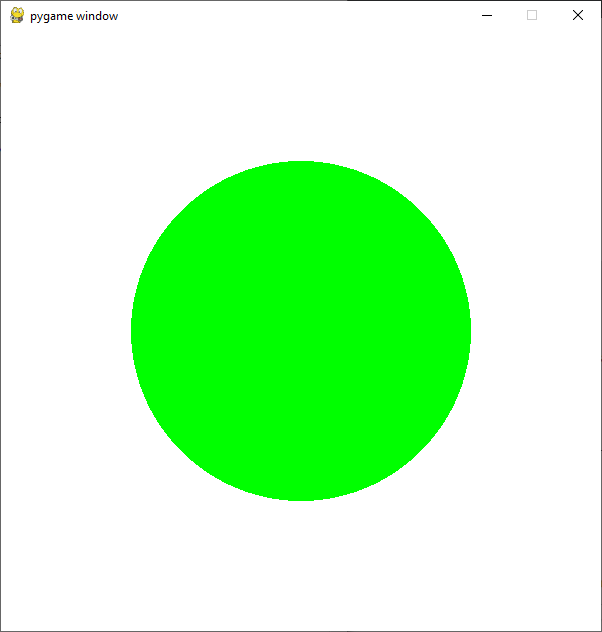Similarly, you can draw other basic shapes using the draw module of pygame.

### Drawing Polygon Shape:

The desired polygon can be drawn using polygon() function.

Syntax: polygon(surface, color, points, width)

Again the approach remains the same only the function and the parameters change.

Example 1: drawing a solid polygon

## Python3

 `# Importing pygame module``import` `pygame``from` `pygame.``locals` `import` `*` `# initiate pygame and give permission``# to use pygame's functionality.``pygame.init()` `# create the display surface object``# of specific dimension.``window ``=` `pygame.display.set_mode((``600``, ``600``))` `# Fill the scree with white color``window.fill((``255``, ``255``, ``255``))` `# Using draw.rect module of``# pygame to draw the outlined polygon``pygame.draw.polygon(window, (``255``, ``0``, ``0``),``                    ``[[``300``, ``300``], [``100``, ``400``],``                     ``[``100``, ``300``]])` `# Draws the surface object to the screen.``pygame.display.update()`

Output :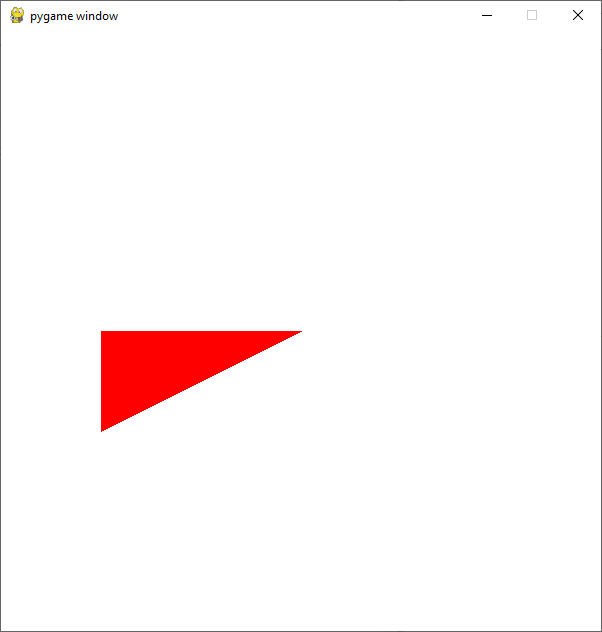Example 2: Drawing a hollow polygon

## Python3

 `# Importing pygame module``import` `pygame``from` `pygame.``locals` `import` `*` `# initiate pygame and give permission``# to use pygame's functionality.``pygame.init()` `# create the display surface object``# of specific dimension.``window ``=` `pygame.display.set_mode((``600``, ``600``))` `# Fill the scree with white color``window.fill((``255``, ``255``, ``255``))` `# Using draw.rect module of``# pygame to draw the outlined polygon``pygame.draw.polygon(window, (``255``, ``0``, ``0``),``                    ``[[``300``, ``300``], [``100``, ``400``],``                     ``[``100``, ``300``]], ``5``)` `# Draws the surface object to the screen.``pygame.display.update()`

Output: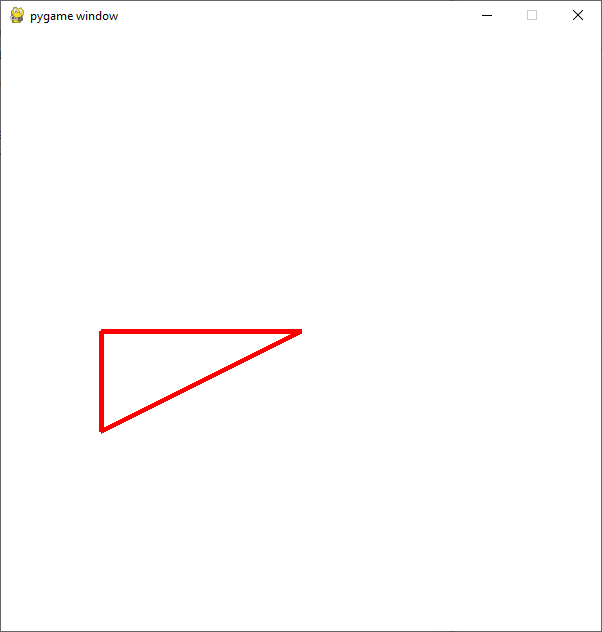### Drawing Line Shape:

A line is the most basic drawing entity and can be drawn in pygame using line() function.

Syntax : pygame.draw.line(surface, color, start_pos, end_pos, width)

Example 1: drawing a line

## Python3

 `# Importing pygame module``import` `pygame``from` `pygame.``locals` `import` `*` `# initiate pygame and give permission``# to use pygame's functionality.``pygame.init()` `# create the display surface object``# of specific dimension.``window ``=` `pygame.display.set_mode((``600``, ``600``))` `# Fill the scree with white color``window.fill((``255``, ``255``, ``255``))` `# Using draw.rect module of``# pygame to draw the line``pygame.draw.line(window, (``0``, ``0``, ``0``),``                 ``[``100``, ``300``],``                 ``[``500``, ``300``], ``5``)` `# Draws the surface object to the screen.``pygame.display.update()`

Output: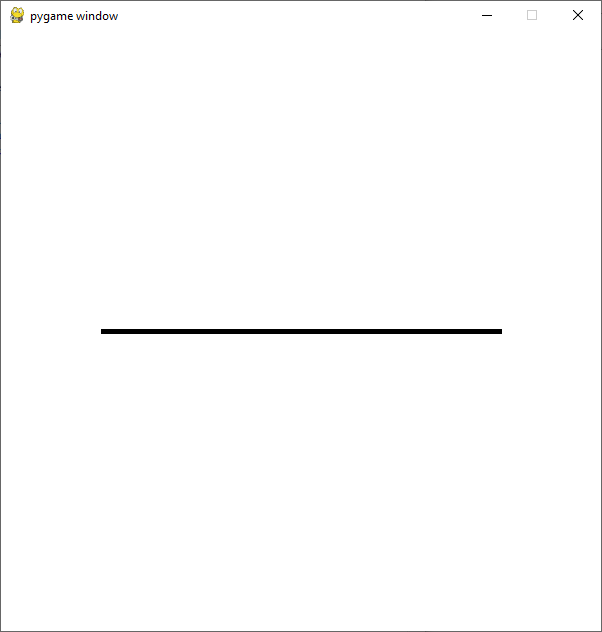## Draw Multiple Shapes:

You can draw multiple shapes on the same surface object. For that, the first required modules are imported and pygame is initialized. Now, create the surface object of a specific dimension using the display.set_mode() method of pygame. Fill the background of the surface object with white color using the fill() function of pygame. Create required shapes are described above. Update the Surface object

Example 1: Drawing a circle inside a rectangle.

## Python3

 `# Importing pygame module``import` `pygame``from` `pygame.``locals` `import` `*` `# initiate pygame and give permission``# to use pygame's functionality.``pygame.init()` `# create the display surface object``# of specific dimension.``window ``=` `pygame.display.set_mode((``600``, ``600``))` `# Fill the scree with white color``window.fill((``255``, ``255``, ``255``))` `# Using draw.rect module of``# pygame to draw the rectangle``pygame.draw.rect(window, (``0``, ``0``, ``255``),``                 ``[``50``, ``200``, ``500``, ``200``])` `# Using draw.rect module of``# pygame to draw the circle inside the rectangle``pygame.draw.circle(window, (``0``, ``255``, ``0``),``                   ``[``300``, ``300``], ``100``)` `# Draws the surface object to the screen.``pygame.display.update()`

Output: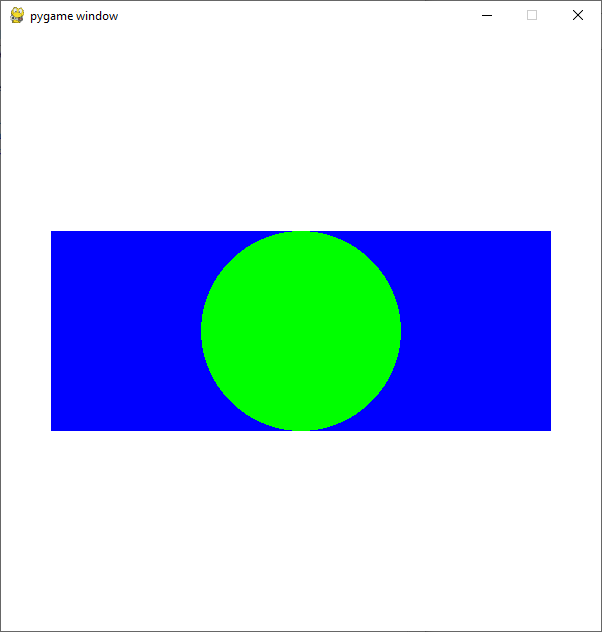Example 2: Drawing rectangles inside one another.

## Python3

 `# Importing pygame module``import` `pygame``from` `pygame.``locals` `import` `*` `# initiate pygame and give permission``# to use pygame's functionality.``pygame.init()` `# create the display surface object``# of specific dimension.``window ``=` `pygame.display.set_mode((``600``, ``600``))` `# Fill the scree with white color``window.fill((``255``, ``255``, ``255``))` `# Creating a list of different rects``rectangle_list ``=` `[pygame.Rect(``50``, ``100``, ``500``, ``200``),``                  ``pygame.Rect(``70``, ``120``, ``460``, ``160``),``                  ``pygame.Rect(``90``, ``140``, ``420``, ``120``),``                  ``pygame.Rect(``110``, ``160``, ``380``, ``80``),``                  ``pygame.Rect(``130``, ``180``, ``340``, ``40``)``                  ``]` `# Creating list of different colors``color_list ``=` `[(``0``,   ``0``,   ``0``),``              ``(``255``, ``255``, ``255``),``              ``(``0``,   ``0``, ``255``),``              ``(``0``, ``255``,   ``0``),``              ``(``255``,   ``0``,   ``0``)``              ``]` `# Creating a variable which we will use``# to iterate over the color_list``color_var ``=` `0` `# Iterating over the rectangle_list using``# for loop``for` `rectangle ``in` `rectangle_list:` `    ``# Drawing the rectangle``    ``# using the draw.rect() method``    ``pygame.draw.rect(window, color_list[color_var],``                     ``rectangle)` `    ``# Increasing the value of color_var``    ``# by 1 after every iteration``    ``color_var ``+``=` `1` `# Draws the surface object to the screen.``pygame.display.update()`

Output :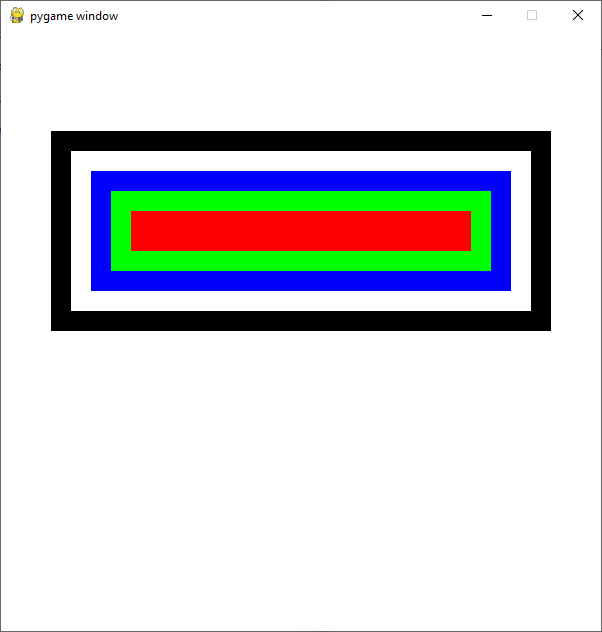## Writing your own drawing functions:

You can easily create your own specialized drawing functions in pygame.

This can be done by following the given procedure. Create a drawing function that will take starting position, width, and height as parameters. Draw required shapes are described above. Call the drawfunction()

## Python3

 `# Importing pygame module``import` `pygame``from` `pygame.``locals` `import` `*` `# Creating Drawing function`  `def` `drawingfunction(x, y, width, height):` `    ``# Creating rectangle using the draw.rect() method``    ``pygame.draw.rect(window, (``0``, ``0``, ``255``), [x, y, width, height])` `    ``# Calculation the center of the circle``    ``circle_x ``=` `width``/``2` `+` `x``    ``circle_y ``=` `height``/``2` `+` `y` `    ``# Calculating the radius of the circle``    ``if` `height < width:``        ``radius ``=` `height``/``2``    ``else``:``        ``radius ``=` `width``/``2` `    ``# Drawing the circle``    ``pygame.draw.circle(window, (``0``, ``255``, ``0``), [circle_x, circle_y], radius)`  `# initiate pygame and give permission``# to use pygame's functionality.``pygame.init()` `# create the display surface object``# of specific dimension.``window ``=` `pygame.display.set_mode((``600``, ``600``))` `# Fill the scree with white color``window.fill((``255``, ``255``, ``255``))` `# Calling the drawing function``drawingfunction(``50``, ``200``, ``500``, ``200``)` `# Draws the surface object to the screen.``pygame.display.update()`

Output:## Drawing shapes with the mouse:

Now let’s see how we can create shapes whenever the user clicks the mouse. We are going to create circles in the next example but you can create any shape you want.

Create a list to store the position of the shape to be drawn. Create a variable to store the color of the shape. Create a variable which we will use to run the while loop and Create a while loop. Iterate over all the events received from pygame.event.get(). If the type of the event is quit then setting the run variable to false. If the type of the event is MOUSEBUTTONDOWN ( this event occurs when a user presses the mouse button) then getting the current position in a variable then appending that position in our circle_positions list. Iterate over all the positions in of the array created using a for a loop. Keep drawing the shape. Update the surface object.

## Python3

 `# Importing pygame module``import` `pygame``from` `pygame.``locals` `import` `*` `# initiate pygame and give permission``# to use pygame's functionality.``pygame.init()` `# create the display surface object``# of specific dimension.``window ``=` `pygame.display.set_mode((``600``, ``600``))` `# Fill the scree with white color``window.fill((``255``, ``255``, ``255``))` `# creating list in which we will store``# the position of the circle``circle_positions ``=` `[]` `# radius of the circle``circle_radius ``=` `60` `# Color of the circle``color ``=` `(``0``, ``0``, ``255``)` `# Creating a variable which we will use``# to run the while loop``run ``=` `True` `# Creating a while loop``while` `run:` `    ``# Iterating over all the events received from``    ``# pygame.event.get()``    ``for` `event ``in` `pygame.event.get():` `        ``# If the type of the event is quit``        ``# then setting the run variable to false``        ``if` `event.``type` `=``=` `QUIT:``            ``run ``=` `False` `        ``# if the type of the event is MOUSEBUTTONDOWN``        ``# then storing the current position``        ``elif` `event.``type` `=``=` `MOUSEBUTTONDOWN:``            ``position ``=` `event.pos``            ``circle_positions.append(position)``            ` `    ``# Using for loop to iterate``    ``# over the circle_positions``    ``# list``    ``for` `position ``in` `circle_positions:` `        ``# Drawing the circle``        ``pygame.draw.circle(window, color, position,``                           ``circle_radius)` `    ``# Draws the surface object to the screen.``    ``pygame.display.update()`

Output:My Personal Notes arrow_drop_up